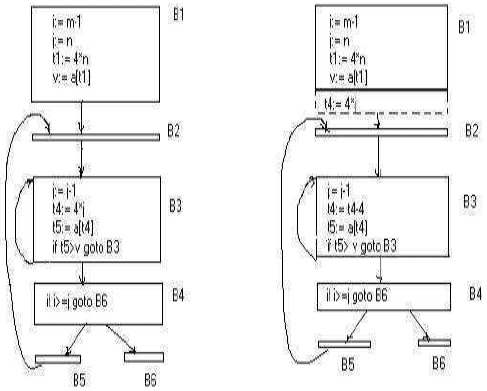Courses

# Optimization Of Basic Blocks Computer Science Engineering (CSE) Notes | EduRev

## Computer Science Engineering (CSE) : Optimization Of Basic Blocks Computer Science Engineering (CSE) Notes | EduRev

The document Optimization Of Basic Blocks Computer Science Engineering (CSE) Notes | EduRev is a part of the Computer Science Engineering (CSE) Course Compiler Design.
All you need of Computer Science Engineering (CSE) at this link: Computer Science Engineering (CSE)

3.  OPTIMIZATION OF BASIC BLOCKSThere are two types of basic block optimizations.
They are :
Structure -Preserving Transformations
Algebraic Transformations

.1 Structure- Preserving Transformations:

The primary Structure-Preserving Transformation on basic blocks are: � Common sub-expression elimination

• Renaming of temporary variables
• Interchange of two independent adjacent statements.

3.2 Common sub-expression elimination:

Common sub expressions need not be computed over and over again. Instead they can be computed once and kept in store from where it’s referenced when encountered again – of course providing the variable values in the expression still remain constant.

Example:
a: =b+c
b: =a-d
c: =b+c
d: =a-d

The 2nd and 4th statements compute the same expression: b+c and a-d

Basic block can be transformed to
a: =b+c
b: =a-d
c: =a
d: =b

It’s possible that a large amount of dead (useless) code may exist in the program. This might be especially caused when introducing variables and procedures as part of construction or error -correction of a program – once declared and defined, one forgets to remove them in case they serve no purpose. Eliminating these will definitely optimize the code. 3.4 Renaming of temporary variables:

•  A statement t:=b+c where t is a temporary name can be changed to u:=b+c where u is another temporary name, and change all uses of t to u.

•   In this we can transform a basic block to its equivalent block called normal-form block.

3.5 Interchange of two independent adjacent statements:

Two statements
t1:=b+c

t2:=x+y

can be interchanged or reordered in its computation in the basic block when value of t1 does not affect the value of t2.

3.6 Algebraic Transformations:

• Algebraic identities represent another important class of optimizations on basic blocks. This includes simplifying expressions or replacing expensive operation by cheaper ones i.e. reduction in strength.

•   Another class of related optimizations is constant folding. Here we evaluate constant expressions at compile time and replace the constant expressions by their values. Thus the expression 2*3.14 would be replaced by 6.28.

•  The relational operators <=, >=, <, >, + and = sometimes generate unexpected common sub expressions.

•  Associative laws may also be applied to expose common sub expressions. For example, if the source code has the assignments

a :=b+c e :=c+d+b

the following intermediate code may be generated:

a :=b+c t :=c+d

e :=t+b

• The  compiler writer  should  examine  the  language carefully to  determine  what rearrangements of  computations  are  permitted;  since computer arithmetic  does  not always obey the algebraic identities of mathematics. Thus, a compiler may evaluate x*y-x*z as x*(y-z) but it may not evaluate a+(b-c) as (a+b)-c.

Offer running on EduRev: Apply code STAYHOME200 to get INR 200 off on our premium plan EduRev Infinity!

## Compiler Design

16 videos|44 docs|29 tests

,

,

,

,

,

,

,

,

,

,

,

,

,

,

,

,

,

,

,

,

,

;